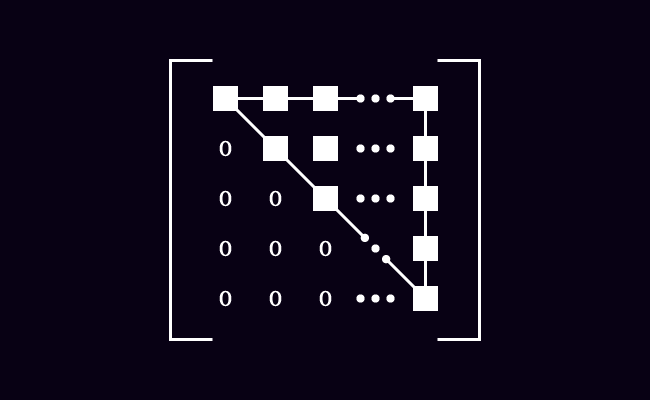Upper Triangular Matrix

Definition

A square matrix whose all elements below the main diagonal are zero, is called an upper triangular matrix.The name of upper triangular matrix describes the internal structure and formation of the matrix.

1. The meaning of upper is above.
2. The meaning of triangular is a triangle shape.
3. The meaning of matrix is a rectangular array, in which elements are arranged in rows and columns.

Combine the meanings of three words, an upper triangular matrix is a special square matrix, in which the elements except below the main diagonal are non-zero elements and the shape of the nonzero elements is a triangle.

An upper triangular matrix can be expressed in the following general form.

$M = \begin{bmatrix} e_{11} & e_{12} & e_{13} & \cdots & e_{1n}\\ 0 & e_{22} & e_{23} & \cdots & e_{2n}\\ 0 & 0 & e_{33} & \cdots & e_{3n}\\ \vdots & \vdots & \vdots & \ddots & \vdots\\ 0 & 0 & 0 & \cdots & e_{mn} \end{bmatrix}$

The elements $e_{21}$, $e_{31}$, $e_{32}$, $e_{41}$, $e_{42}$, $e_{43}$ and etc. are zero but the remaining elements are nonzero elements.

The general form matrix can also be expressed in compact form.

$M = {\begin{bmatrix} e_ij} \end{bmatrix}}_m \times n}$

There is a condition in the case of an upper triangular matrix. The elements are zero if $i > j$ and they are elements under the main diagonal of the matrix. The remaining elements are non-zero elements and form a triangle shape.

In other words, $e_{ij} = 0$ if $i > j$.

Example

The following examples understand you the upper triangular matrices.

$A = \begin{bmatrix} 2 & -4\\ 0 & 3 \end{bmatrix}$

$A$ is a square matrix and also an upper triangular matrix of order $2 \times 2$. In this case the element $e_{21}$ is zero and other elements are nonzero and formed a triangle shape.

$B = \begin{bmatrix} 1 & 5 & 6\\ 0 & 3 & 7\\ 0 & 0 & 4 \end{bmatrix}$

$B$ is an upper triangular matrix of order $3 \times 3$ and the elements $e_{21}$, $e_{31}$ and $e_{32}$ are zero and the shape of nonzero elements is a triangle.

$C = \begin{bmatrix} 4 & 2 & -3 & 9\\ 0 & -1 & 6 & -5\\ 0 & 0 & 8 & 3\\ 0 & 0 & 0 & 5\\ \end{bmatrix}$

$C$ is an example for an upper triangular matrix. In this square matrix, the elements $e_{21}$, $e_{31}$, $e_{32}$, $e_{41}$, $e_{42}$ and $e_{43}$ are zero and the remaining elements are nonzero and the arrangement of the nonzero elements is a triangle shape.

Latest Math Topics
Latest Math Problems
Email subscription
Math Doubts is a best place to learn mathematics and from basics to advanced scientific level for students, teachers and researchers. Know more